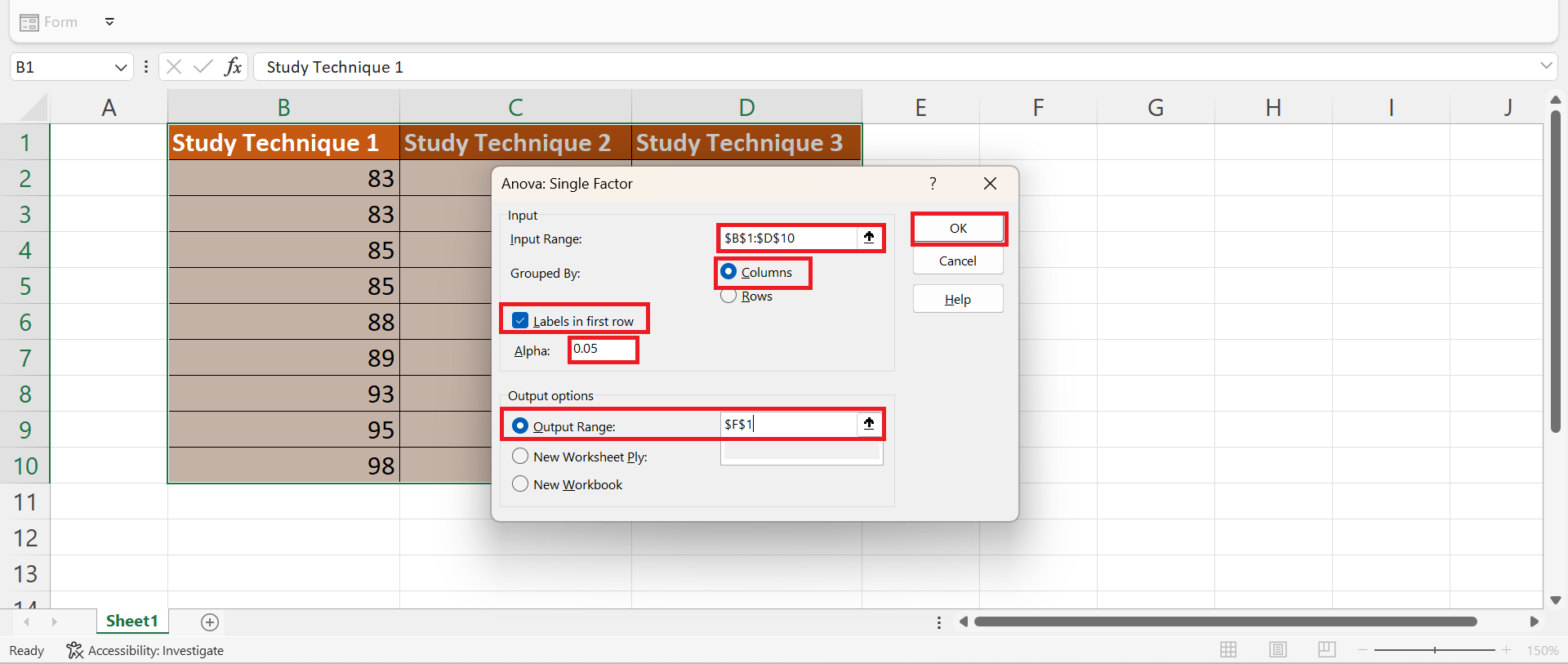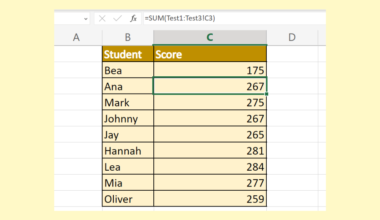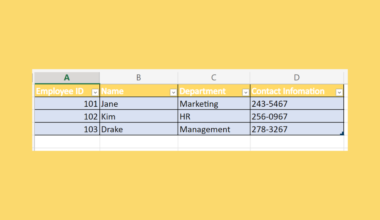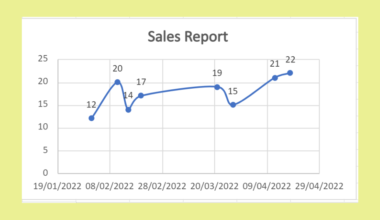# How to Perform a Bonferroni Correction in Excel

This guide will explain how to perform a Bonferroni correction in Excel.

Since it has several built-in tools and functions, Excel is popular for different situations and purposes. Moreover, we can easily perform complex and long data analysis techniques in Excel.

In this guide, we will focus on learning how to perform a Bonferroni correction in Excel. So the Bonferroni correction is a statistical technique that allows us to calculate the correct alpha level. And we would need to use the adjusted alpha level to control the probability of having a type I error.

This all sounds complicated. However, we can easily perform a Bonferroni correction in Excel using the several built-in functions and tools it has. Specifically, we will use the data analysis tool and the `T.TEST` function to perform a Bonferroni correction.

Let’s take a sample scenario wherein we need to perform a Bonferroni correction in Excel.

Suppose you are a teacher who is recording the test scores of the students. And the students are using one out of three different study techniques while preparing for the test. Then, we performed a one-way ANOVA to determine whether the mean test scores were equal across the three groups.

Based on the results, at least one group of students is different from the rest. Since you want to identify which group is different, you performed a Bonferroni correction to compare the three groups.

Before we move on to a real example of performing a Bonferroni correction in Excel, let’s first understand how to write the `T.TEST` function.

## The Anatomy of the T.TEST Function

The syntax or the way we write the `T.TEST` function is as follows:

`=T.TEST(array1, array2, tails, type)`

Let’s take apart this formula and understand what each term means:

• = the equal sign is how we activate any function in Excel.
• T.TEST() is our `T.TEST` function. And this function is used to return the probability associated with a student’s t-test. Moreover, we can use this function to determine if two samples are likely to have come from the same two populations with the same mean.
• array1 is a required argument. So this refers to the first data set we want to perform the t-test.
• array2 is another required argument. And this refers to the second data set we want to perform the t-test.
• tails is also a required argument. So this refers to the specific number of distribution tails to return. When we input 1, the function will return a one-tailed distribution. When we input 2, the function will return a two-tailed distribution.
• type is another required argument. And this refers to the kind of t-test we want to perform. If we want a paired t-test, we can input 1. If we want a two-sample equal variance or homoscedastic, we can input 2. If we want a two-sample unequal variance, we can input 3.

Great! Now we can dive into a real example of performing a Bonferroni correction in Excel.

## A Real Example of Performing a Bonferroni Correction in Excel

Let’s say we have a data set about students’ test scores. However, the students used three different study techniques. So we have three columns showing each student’s scores under a specific study technique. So our initial data set would look like this:Given our data set, we want to perform a one-way ANOVA to identify whether the mean test scores are equal across all three groups. Luckily, we can easily perform a one-way ANOVA in Excel using the data analysis tool.

Furthermore, a one-way ANOVA has a null hypothesis and an alternative hypothesis. So the null hypothesis states that all three group means are equal, while the alternative hypothesis states that at least one group mean is different from the rest.

Since the result of our one-way ANOVA returned a p-value less than 0.05, we have enough evidence to reject the null hypothesis which means one group has a mean score different from the others.

To identify which group has a different mean score, we need to perform multiple comparisons using the Bonferroni correction. To do this, we can calculate the adjusted alpha level using the formula `αnew = αoriginal / n`

In the formula, αoriginal refers to the original alpha level, and n is the total number of comparisons being performed.

For our example, we will have a total of three comparisons which are study technique 1 versus study technique 2, study technique 1 versus study technique 3, and study technique 2 and study technique 3.

Next, the Bonferroni correction will give us the adjusted alpha level we need to use for each test. Afterward, we can utilize the `T.TEST` function to compare the means between each group.

Then, we can simply find the p-value that is less than the Bonferroni adjusted alpha level value from the three comparisons. Thus, we can conclude which study technique group is statistically significantly different in mean test scores.

So our final data set would look like this:Amazing! Now we can dive into the steps of how to perform a Bonferroni correction in Excel.

## How to Perform a Bonferroni Correction in Excel

In this section, we will explain the step-by-step process of how to perform a Bonferroni correction in Excel. Additionally, each step has detailed instructions and pictures to guide you through the process.

To apply this method to your work, simply follow the steps below.

1. Firstly, we will perform a one-way ANOVA using the data analysis tool. To do this, we will select the entire data set and go to the Data tab. Then, we will click Data Analysis.2. In the Data Analysis window, we will select Anova: Single Factor. Lastly, we will click OK.3. Next, we will select Columns under the Grouped By section. Afterward, we will check the box for Labels in the first row and input the value “0.05” for Alpha. Then, we will select Output Range and select a new cell location to display the results. Lastly, we will click OK to apply all the changes.4. After that, we will first calculate the adjusted alpha level value using the formula `αnew = αoriginal / n`. To do this, we can simply type in the formula “=0.05/3”. Lastly, we will press the Enter key to return the result.5. Then, we will use the `T.TEST` function to compare the means. So we will first perform the first comparison of study technique 1 versus study technique 2. To do this, we will input the formula “=T.TEST(B2:B10, C2:C10, 2, 2)”.6. Next, we will do a comparison of study technique 1 versus study technique 3. To do this, we will type in the formula “=T.TEST(B2:B9, D2:D10, 2, 2, 2)”.7. Lastly, we will do a comparison of study technique 2 versus study technique 3. To do this, we will input the formula “=T.TEST(C2:C10, D2:D10, 2, 2, 2)”.8. And tada! We have successfully performed a Bonferroni correction in Excel.And that’s pretty much it! We have successfully explained how to perform a Bonferroni correction in Excel. Now you can apply this method whenever you need it.

Are you interested in learning more about what Excel can do? You can now use the `T.TEST` function and the various other Microsoft Excel formulas available to create great worksheets that work for you. Make sure to subscribe to our newsletter to be the first to know about the latest guides and tutorials from us.Our goal this year is to create lots of rich, bite-sized tutorials for Google Sheets users like you. If you liked this one, you'll love what we are working on! Readers receive ✨ early access ✨ to new content.

##### You May Also Like## How to Sum Across Multiple Sheets in Excel

This guide will explain how to sum across multiple sheets in Excel using the SUM function and 3D…## How to Construct a Prediction Interval in Excel

This guide will explain how to construct a prediction interval in Excel. The prediction interval uses historical data…## How to Create a Library Database in Excel

This guide will explain how to create a library database in Excel using the table feature.  Excel is…## How to Create a Gauge Chart in Excel

The gauge chart in Excel is useful when you want to create an eye-catching visualization for a certain…